Courses
Courses for Kids
Free study material
Free LIVE classes
More

# Operation in MathematicsLIVE
Join Vedantu’s FREE Mastercalss

## Exploring Four Fundamental Operations of Mathematics

The fundamentals of mathematics are arithmetic operations. The basic operations that can be performed on relations are addition, subtraction, multiplication, and division make up most of them. We use them nearly constantly throughout the day. Daily, we use mathematical operations to calculate overall business revenue and expenses, create monthly or yearly budgets, measure distances, and more. For example, we use them to calculate the overall number of homework questions, time, money, the number of chocolates we ate, the total number of points we received across all subjects, etc. In this article, we will learn about these basic arithmetic operations.

## What are the Operations in Mathematics?

Calculating a value using operands and a math operator is called performing a mathematical "operation." The math operator's symbol has predetermined rules that must be applied to the operands or numbers.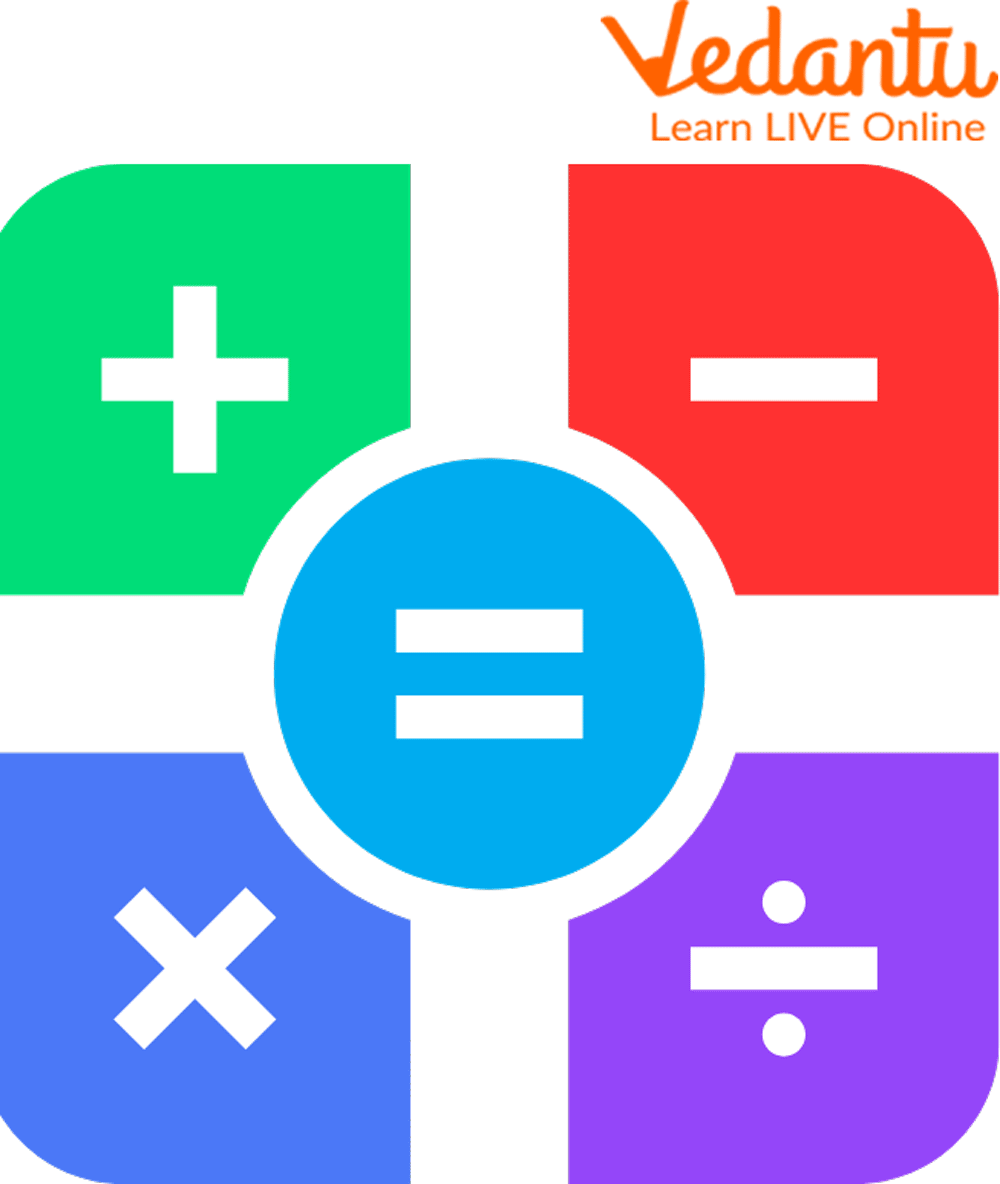Mathematical Operations

A set of numbers and operations make up a mathematical expression. The components of a mathematical expression that operates are:

1. Operands: Operands are the numerical values that are used in operation. Different words are given to the operands depending on the type of operation.

2. Operator: An operator is a symbol used to represent a mathematical operation.

## Basic Arithmetic Operations

The arithmetic operators carry out the addition, subtraction, multiplication, division, exponentiation, and modulus operations. The four fundamental arithmetic operations in mathematics for all real numbers are addition, subtraction, multiplication, and division. The operators for the following operations are as follows:

2. − represents subtraction

3. × represents multiplication

4. ÷ represents division

5. = represents equal to, indicates the equivalence; that is, the left-hand side value is equal to the right-hand side value.

We have already discussed the four fundamental operations on the whole numbers. Let’s understand their application and significance one by one.

Addition refers to combining two or more figures (or objects) to create a new sum.

The addition is known as sum, plus, increase, and total.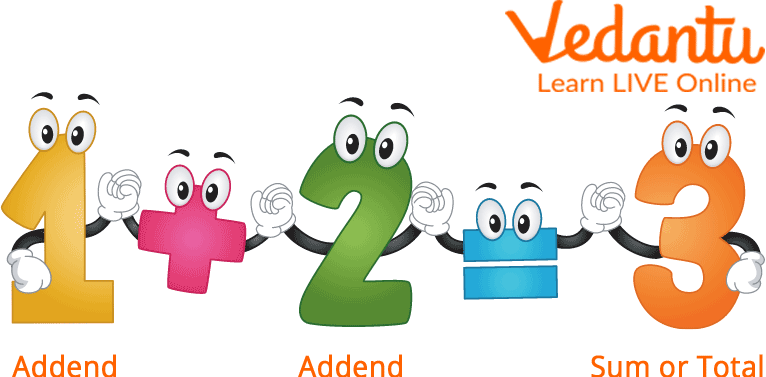## What is Subtraction (-)?

The subtraction arithmetic operation shows the difference between two numbers. The numbers in a subtraction fact have the following names: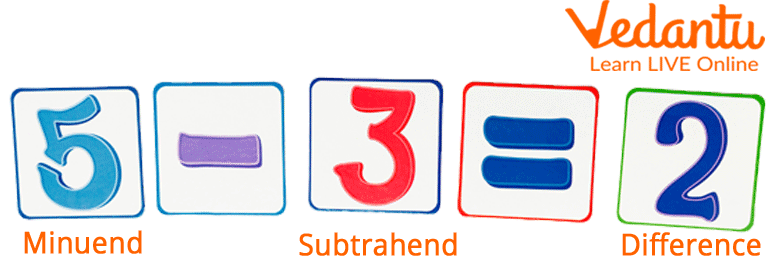Subtraction

Here, minuend is the number from which the other number is subtracted, and subtrahend is the number that is subtracted from the minuend. The symbol (-) indicates the subtraction operation, and the difference indicates the result.

## What is Multiplication?

Multiplication is one of the four basic mathematical functions, along with addition, subtraction, and division. Multiply in mathematics refers to the repetitive addition of sets of identical sizes. The multiplier is the number of equivalent groupings, and the multiplicand is the number of things contained in each group.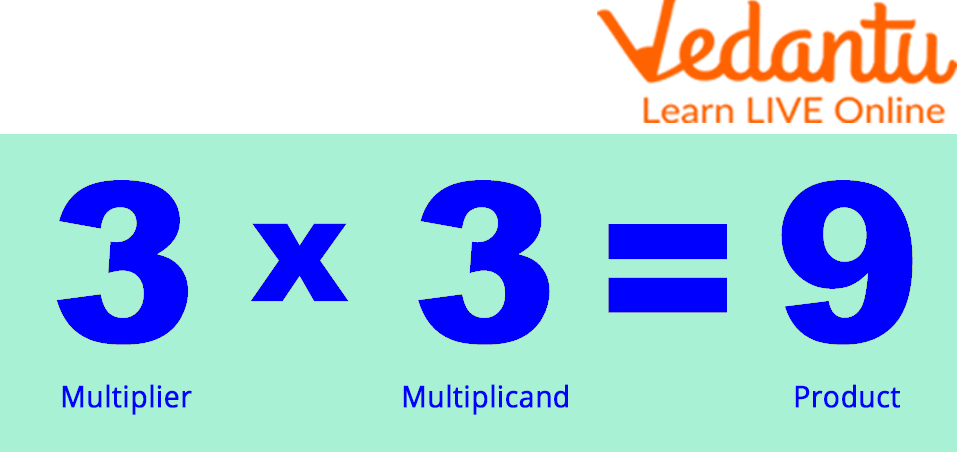Multiplication

## What is Division?

The division is one of the four basic mathematical functions, along with addition, subtraction, and multiplication. The division divides a larger group into smaller groups so that each group contains an equal number of things.

Two fundamental division symbols represent the division of two numbers. These are ÷ and /.

The division comprises the dividend, the divisor, the quotient, and the remainder. The names of the phrases connected with the division process are referred to as division parts. Let's examine the division example presented below better to grasp the significance of these four division components.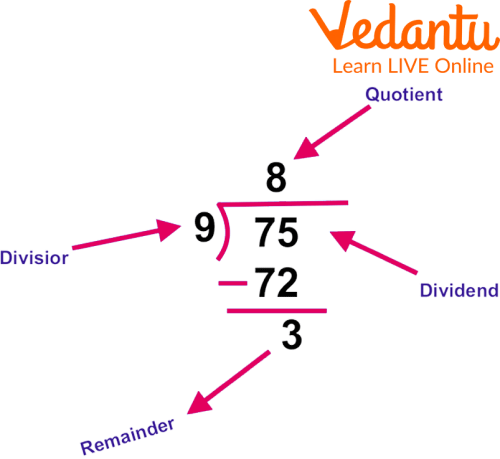Division

Dividend = Divisor $\times$ Quotient $+$ Remainder

$75=9 \times 8+3$

Here, the values of a divisor, dividend, quotient, and remainder are obtained by dividing 75 by 9.

## Solved Examples

Example 1: Sally and Jimmy each collected 24 and 19 eggshells, respectively. How many eggshells did they collect altogether?

Ans: Given

Number of eggshells collected by Sally = 24

Number of eggshells collected by Jimmy = 19

As a result, they both collected a total of 24+19 = 43 eggshells.

Example 2: If a box contains 88 balls. There are 35 blue balls. How many non-blue balls are there in the box?

Ans: Given the total number of balls = 88

Number of blue balls=35

The number of Non-blue balls =88-35=53.

Hence, 53 non-blue balls are there in the box.

Example 3: There are 100 sheets of paper in each of the 14 folders. What will be the total of sheets of paper there?

Ans: Given 14 folders, each with 100 sheets.

Therefore, the total number of sheets = $14 \times 100=1400$

Example 4: To make a glass of lemon juice, Daisy needs 5 lemons. The number of glasses of lemon juice Nancy can produce if she has 155 lemons.

Ans: One glass requires 5 lemons.

Total number of Lemons=155

The number of glasses of lemon juice = $\dfrac{155}{5}=31$ glasses.

## Practice Problems

Q 1. The auditorium has 194 blue seats and 256 yellow seats. What is the total number of seats there in the auditorium?

Ans: 450 Seats

Q 2. Plain paper in 876 sheets is available to Rakesh. To write a book, he uses 455 sheets. How many plain sheets are still with him?

Ans: 421 sheets

Q 3. One packet carries 12 notebooks. How many notebooks are in 72 of these packets?

Ans: 864 notebooks

## Summary

For building operations in mathematics, that is what we call mathematics. So, it is very important to learn how to perform these operations. The four building blocks of mathematics are addition, subtraction, multiplication, and division. In mathematics, the sum of two numbers is the outcome of their addition. Subtracting one number's value from another is known as subtraction. Multiplication can be conceptualized as repeated addition. The opposite of multiplication is frequently used to describe division. At the end of the article, we have added the practice word problem based on the operation of mathematics. After going through the article, try the practice questions and check your understanding.

Last updated date: 21st Sep 2023
Total views: 91.8k
Views today: 2.91k

## FAQs on Operation in Mathematics

1. What are Addition and Subtraction Facts?

The following is a list of some of the basic facts about addition and subtraction:

• Any number is equal to itself when 0 is added or subtracted. To illustrate, 6 + 0 = 6.

• Any number's successor is obtained by adding 1, and any number's predecessor is obtained by deducting 1 from it.

• Inverse operations include addition and subtraction.

2. In an equation, what is the order of operations?

Teachers in the United States drill the acronym PEMDAS into their students to help them remember this order of operations: parentheses, exponents, multiplication, division, addition, and subtraction. So, the order of operations is a rule that specifies the right procedure for evaluating a mathematical equation. Parentheses, Exponents, Multiplication, and Division (from Left to Right), Addition, and Subtraction are the steps we can remember using PEMDAS (from left to right).

3. When should you use BODMAS?

When completing a mathematical number sentence including many operations, BODMAS assists you in determining the order to execute them. Anything in brackets should be completed first, followed by order, division or multiplication, and addition or subtraction.

Thus, BODMAS enables you to choose which order to carry out the various operations in a mathematical number phrase, including numerous separate operations. Any division or multiplication should follow the order, any items in brackets, and any addition or subtraction.